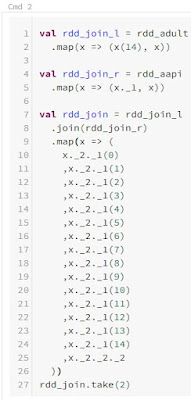# Breaking BI

Today, we’re going to continue talking about RDDs, Data Frames and Datasets in Azure Databricks.

Today, we’re going to continue talking about RDDs, Data Frames and Datasets in Azure Databricks.  If you haven’t read the previous posts in this series, Introduction, Cluser Creation, Notebooks, Databricks File System (DBFS), Hive (SQL) Database and RDDs, Data Frames and Dataset (Part 1, Part 2), they may provide some useful context.  If you are new to this material, it is especially important that you read through the previous posts (Part 1, Part 2), as this post will build directly on what was discussed during the previous post.  You can find the files from this post in our GitHub Repository.  Let’s move on to the core of this post, RDDs, Data Frames and Datasets.

Before we begin, the following code block will create all of the necessary objects from the previous posts (Part 1, Part 2).

<CODE START>

import org.apache.spark.sql.functions._

.filter(f => f != “”)
.map(l => l.split(“, “))

.option(“inferSchema”, “true”)
“age”
,”workclass”
,”fnlwgt”
,”education”
,”educationnum”
,”maritalstatus”
,”occupation”
,”relationship”
,”race”
,”sex”
,”capitalgain”
,”capitalloss”
,”hoursperweek”
,”nativecountry”
,”income”
)

age: Int
,workclass: String
,fnlwgt: Double
,education: String
,educationnum: Double
,maritalstatus: String
,occupation: String
,relationship: String
,race: String
,sex: String
,capitalgain: Double
,capitalloss: Double
,hoursperweek: Double
,nativecountry: String
,income: String
)

.map(x => (x(0), x(14)))

.select(“age”, “income”)

age: Int
,income: String
)

.select(“age”, “income”)

age: Int
,income: String
)

.map(x => (x.age, x.income))
.withColumnRenamed(“_1”, “age”)
.withColumnRenamed(“_2”, “income”)

.map(x => (x(0), x(0).toInt * 12))

.withColumn(“ageinmonths”, \$”age” * 12)
.select(“age”, “ageinmonths”)

age: Int
,ageinmonths: Int
)

.withColumn(“ageinmonths”, \$”age” * 12)
.select(“age”, “ageinmonths”)

.filter(x => x(14) == “<=50K”)

.filter(ltrim(\$”income”) === “<=50K”)

.filter(ltrim(\$”income”) === “<=50K”)

.map(x => x(10).toDouble)
.reduce((a, b) => a + b)

.agg(sum(“capitalgain”))
.first()
.getDouble(0)

.agg(sum(“capitalgain”))
.first()
.getDouble(0)

.map(x => x(0).toInt)
.aggregate((0, 0))(
(w, age) => (w._1 + age, w._2 + 1)
,(a, b) => (a._1 + b._1, a._2 + b._2)
)
val rdd_avg = rdd_avg_tup._1.toDouble / rdd_avg_tup._2

.agg(avg(“age”))
.first()
.getDouble(0)

.agg(avg(“age”))
.first()
.getDouble(0)

val ttuple = (1,2,3)
val tarray = Array(1,2,3)

.map(x => (x(14), x(0).toInt))
.aggregateByKey((0, 0))(
(w, age) => (w._1 + age, w._2 + 1)
,(a, b) => (a._1 + b._1, a._2 + b._2)
)
.map(x => (x._1, x._2._1.toDouble / x._2._2))

.groupBy(“income”)
.agg(avg(“age”))

income: String
,avgage: Double
)

.groupBy(“income”)
.agg(avg(“age”))
.withColumnRenamed(“avg(age)”, “avgage”)

<CODE END>

In the previous posts (Part 1, Part 2), we’ve looked at a number of basic transformations and aggregations.  Now, let’s take a look at joining objects together.  In the previous post, we created objects containing the average age per income level.  Let’s try joining those into the original objects.Join RDD CodeJoin RDD Output

<CODE START>

.map(x => (x(14), x))

val rdd_join_r = rdd_aapi
.map(x => (x._1, x))

val rdd_join = rdd_join_l
.join(rdd_join_r)
.map(x => (
x._2._1(0)
,x._2._1(1)
,x._2._1(2)
,x._2._1(3)
,x._2._1(4)
,x._2._1(5)
,x._2._1(6)
,x._2._1(7)
,x._2._1(8)
,x._2._1(9)
,x._2._1(10)
,x._2._1(11)
,x._2._1(12)
,x._2._1(13)
,x._2._1(14)
,x._2._2._2
))

rdd_join.take(2)

<CODE END>Join Data Frame

<CODE START>

val df_join_r = df_aapi
.withColumnRenamed(“income”, “rincome”)

.join(
df_join_r
,”inner”
)
.drop(“rincome”)

df_join.show(2)

<CODE END>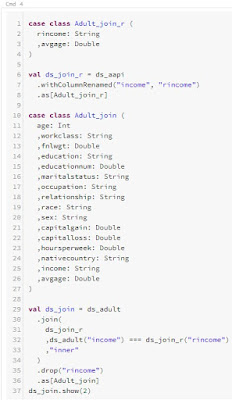Join Dataset Code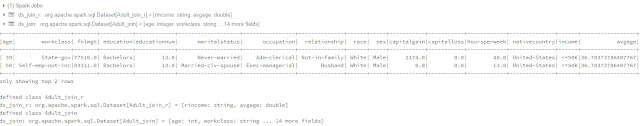Join Dataset Output

<CODE START>

rincome: String
,avgage: Double
)

val ds_join_r = ds_aapi
.withColumnRenamed(“income”, “rincome”)

age: Int
,workclass: String
,fnlwgt: Double
,education: String
,educationnum: Double
,maritalstatus: String
,occupation: String
,relationship: String
,race: String
,sex: String
,capitalgain: Double
,capitalloss: Double
,hoursperweek: Double
,nativecountry: String
,income: String
,avgage: Double
)

.join(
ds_join_r
,”inner”
)
.drop(“rincome”)

ds_join.show(2)

<CODE END>

We can make use of the built-in .join() function for RDDs.  Similar to the .aggregateByKey() function we saw in the previous post, the .join() function for RDDs requires a 2-element tuple, with the first element being the key and the second element being the value.  So, we need to use the .map() function to restructure our RDDs to store the keys in the first element and the original array/tuple in the second element.  After the join, we end up with an awkward nested structure of arrays and tuples that we need to restructure using another .map() function, leading to a lengthy code snippet.

Data Frames and Datasets can also make use their own .join() function, with the ability to define a binary join condition using the === notation we saw in the previous post.  Since Data Frames and Datasets have column names, we need to rename the key column in the right Data Frame or Dataset using the .withColumnRenamed() function to avoid duplicate column names.  After that, we can drop the right key using the .drop() function.  Once again, we see that the primary difference when working with Datasets is that we need to explicitly create a case class for each new Dataset we create.

In this case, we wanted to perform an inner join.  However, we can also perform other join types using the leftOuterJoin(), rightOuterJoin() and fullOuterJoin() functions for RDDs.  Data Frames and Datasets have a bit more flexibility on this front, allowing us to pass in the following join types as strings: inner, cross, outer, full, full_outer, left, left_outer, right, right_outer, left_semi, left_anti.

Next, let’s try sorting our objects by age.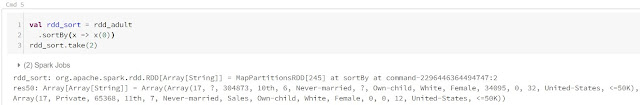Sort RDD

<CODE START>

.sortBy(x => x(0))

rdd_sort.take(2)

<CODE END>Sort Data Frame

<CODE START>

.orderBy(“age”)

df_sort.show(2)

<CODE END>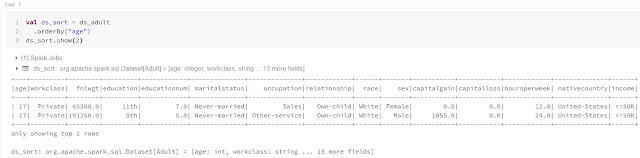Sort Dataset

<CODE START>

.orderBy(“age”)

ds_sort.show(2)

<CODE END>

We were able to leverage the sortBy() function to sort the RDD.  All we needed to provide was an anonymous function that returns the values of interest.  The Data Frame and Dataset were similarly simple, using the .orderBy() function and a column name.  Since the structure of the Dataset didn’t change, we didn’t need create a new case class for it.

Although, sorting by age alone doesn’t produce repeatable results, as age is not unique.  So, let’s sort by age and workclass.Sort RDD 2

<CODE START>

.sortBy(x => (x(0), x(1)))

rdd_sort.take(2)

<CODE END>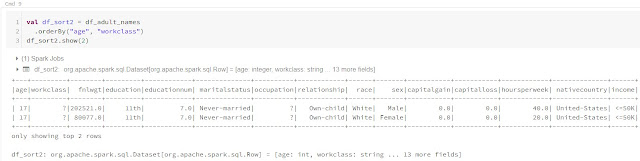Sort Data Frame 2

<CODE START>

.orderBy(“age”, “workclass”)

df_sort2.show(2)

<CODE END>Sort Dataset 2

<CODE START>

.orderBy(“age”, “workclass”)

ds_sort2.show(2)

<CODE END>

We see that this is equally simple.  For RDDs, we need to use a tuple instead of a single value.  For Data Frames and Datasets, we need to pass additional column into the orderBy() function.

That’s all of the functionality that we wanted to cover in this mini-series comparing the data storage objects.  Using the tools that we’ve found over these three posts, the majority of data transformation problems are within our grasp.  We’ve seen that, for the most part, Data Frames and Datasets provide much simpler and more readable code because of their SQL-esque nature.  But, there’s still one more topic we need to cover, Performance.  Stay tuned for the next post where we’ll ramp up the data volume to see if there are any substantial performance impacts of using these objects.  Thanks for reading.  We hope you found this informative.